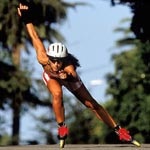Resistance of gravity in skating (roller or ice)

For a skater, the gravity affects the speed with a negative effect for ascent or positive for descent. On a flat way, gravity is not negligible or zero unlike other sports (cycling for example).

Newton's law

The Newton's law determines the role of the hearth attraction. The gravity resistance Rg depends on the slope Pt (slope calculation) and the total weight, i.e. the total mass Mt (skater plus equipment) and the acceleration of gravity g. Obviously, this resistance is zero on a flat way.

Style

The gesture to move forward with skates consists of alternated and repeated thrusts. It introduces a vertical oscillating movement of the gravity center of the skater's body. The associated resistance Rs depends (as above) on the total weight and eventual slope. According to the skating style, it is possible to evaluate the resistance with a coefficient Cs.

 We can consider: Cs = 0.010 for "irregular" skating (novice, sprint, etc.) Cs = 0.008 for "dynamic" skating Cs = 0.006 for "fluid" skating Cs = 0.004 for "economic" skatingN.B. Cs represents only the gesture cost for gravity and should not be confused with the global gesture efficiency.

Calculation formula

In summary, the mathematic formula allowing calculation is:
Rg = Mt g Sin(Arctan(Pt))
Rs = Mt g Cs Cos(Arctan(Pt))

Calculation of the skating power

J.L